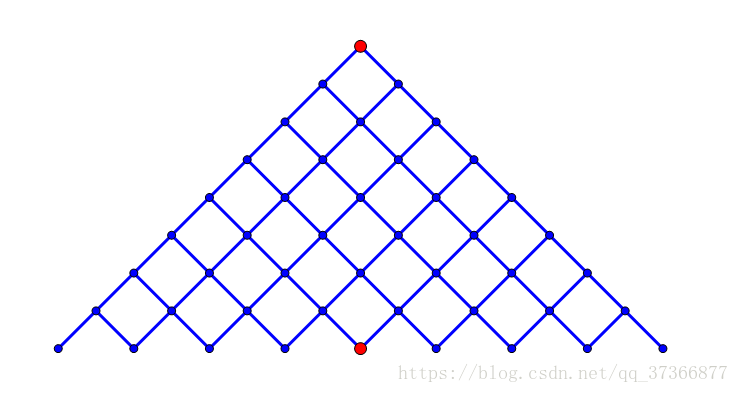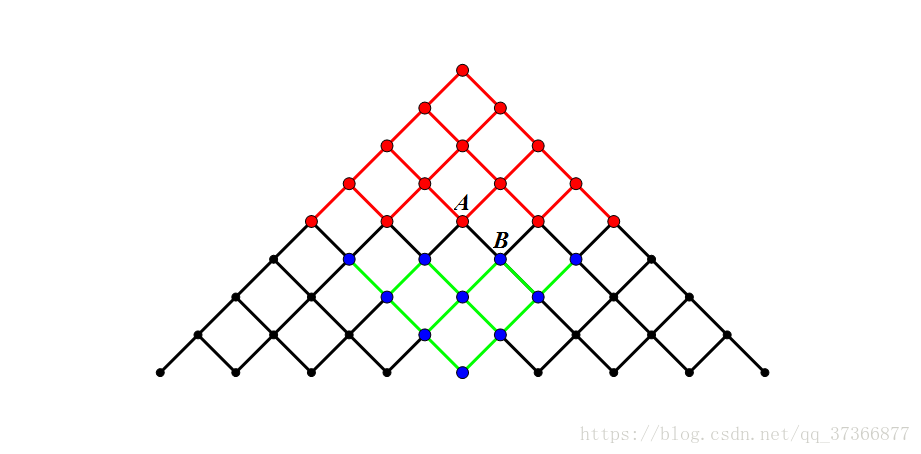# meet in the middle——折半查找算法

## meet in the middle 简介## 例题

### 494. 目标和 - 力扣（LeetCode）

#### 题目描述

• 例如，`nums = [2, 1]` ，可以在 `2` 之前添加 `'+'` ，在 `1` 之前添加 `'-'` ，然后串联起来得到表达式 `"+2-1"`

```输入：nums = [1,1,1,1,1], target = 3

-1 + 1 + 1 + 1 + 1 = 3
+1 - 1 + 1 + 1 + 1 = 3
+1 + 1 - 1 + 1 + 1 = 3
+1 + 1 + 1 - 1 + 1 = 3
+1 + 1 + 1 + 1 - 1 = 3
```

```输入：nums = , target = 1

```

• `1 <= nums.length <= 20`
• `0 <= nums[i] <= 1000`
• `0 <= sum(nums[i]) <= 1000`
• `-1000 <= target <= 1000`

### 805. 数组的均值分割 - 力扣（LeetCode）

#### 题目描述

```输入: nums = [1,2,3,4,5,6,7,8]

```

```输入: nums = [3,1]

```

• `1 <= nums.length <= 30`
• `0 <= nums[i] <= 104`